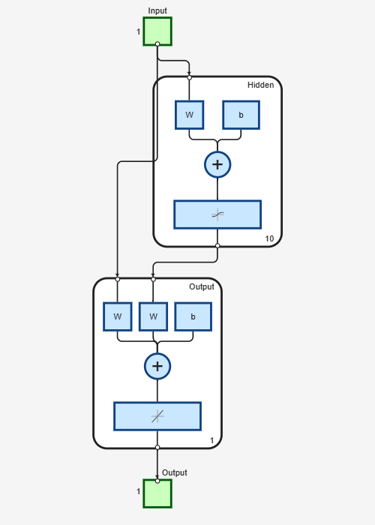## Syntax

``net = cascadeforwardnet(hiddenSizes,trainFcn)``

## Description

example

````net = cascadeforwardnet(hiddenSizes,trainFcn)` returns a cascade-forward neural network with a hidden layer size of `hiddenSizes` and training function, specified by `trainFcn`.Cascade-forward networks are similar to feed-forward networks, but include a connection from the input and every previous layer to following layers.As with feed-forward networks, a two-or more layer cascade-network can learn any finite input-output relationship arbitrarily well given enough hidden neurons.```

## Examples

collapse all

This example shows how to use a cascade-forward neural network to solve a simple problem.

`[x,t] = simplefit_dataset;`

The 1-by-94 matrix `x` contains the input values and the 1-by-94 matrix `t` contains the associated target output values.

Construct a cascade-forward network with one hidden layer of size 10.

`net = cascadeforwardnet(10);`

Train the network `net` using the training data.

`net = train(net,x,t);`View the trained network.

`view(net)`Estimate the targets using the trained network.

`y = net(x);`

Assess the performance of the trained network. The default performance function is mean squared error.

`perf = perform(net,y,t)`
```perf = 1.9372e-05 ```

## Input Arguments

collapse all

Size of the hidden layers in the network, specified as a row vector. The length of the vector determines the number of hidden layers in the network.

Example: For example, you can specify a network with 3 hidden layers, where the first hidden layer size is 10, the second is 8, and the third is 5 as follows: `[10,8,5]`

The input and output sizes are set to zero. The software adjusts the sizes of these during training according to the training data.

Data Types: `single` | `double`

Training function name, specified as one of the following.

Training FunctionAlgorithm
`'trainlm'`

Levenberg-Marquardt

`'trainbr'`

Bayesian Regularization

`'trainbfg'`

BFGS Quasi-Newton

`'trainrp'`

Resilient Backpropagation

`'trainscg'`

`'traincgb'`

`'traincgf'`

`'traincgp'`

`'trainoss'`

One Step Secant

`'traingdx'`

`'traingdm'`

`'traingd'`

Example: For example, you can specify the variable learning rate gradient descent algorithm as the training algorithm as follows: `'traingdx'`

For more information on the training functions, see Train and Apply Multilayer Shallow Neural Networks and Choose a Multilayer Neural Network Training Function.

Data Types: `char`

## Output Arguments

collapse all

Cascade-forward neural network, returned as a `network` object.

## Version History

Introduced in R2010b